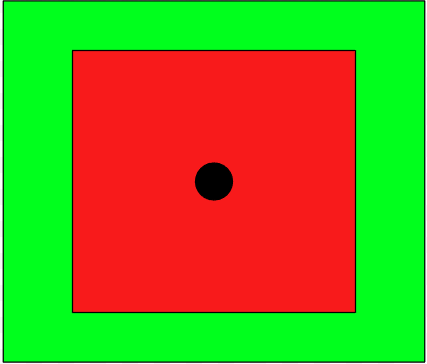# How do I make objects not spawn inside a certain radius (Inside of a random spawning radius)

(SCROLL DOWN FOR ANSWER) I want objects to NOT spawn inside of a certain radius (Players View which is +/-20 on x & z). How would I make it exclude this area. Since the player will move it should not be a fixed position but should move with the player. Please TRY to keep answers somewhat simple if that’s possible!
(Also, please tell me how I could make the spawn radius follow the player so the zombies can spawn closer to the player and not 400 meters out)

``````    //Array of different zombies
public GameObject[] zombiePrefabs;

//Spawn Timer
float startSpawnDelay = 3.0f;
float spawnTimeDelay = Random.Range(1.5f, 3.5f);
//Spawn Timer---------------------------------------
InvokeRepeating("SpawnRandomZombie", startSpawnDelay, spawnTimeDelay);
}

void SpawnRandomZombie()
{
if (detectCollisionsScript.gameOver != true)
{
int zombieIndex = Random.Range(0, zombiePrefabs.Length);
Instantiate(zombiePrefabs[zombieIndex], RandomSpawnPos(), zombiePrefabs[zombieIndex].transform.rotation);
}
}

Vector3 RandomSpawnPos()
{
float spawnPosX = 20f;
float spawnPosZ = 20f;
float spawnPosY = 0;
Vector3 spawnPos = new(Random.Range(-spawnPosX, spawnPosX), spawnPosY, Random.Range(-spawnPosZ, spawnPosZ));
Vector3 playerPosDifference = spawnPos + player.transform.position;
return playerPosDifference; //Here is where I'm having trouble with spawning the zombies
}

``````

## Diagram of what it should beGreen is where the enemies can spawn (+/- 23 x/z)
Red is where enemies can NOT spawn (+/- 20 x/z)

I did end up fixing this so if anyone if the future is reading this, this is the code I used (It is quite long and could be optimized) :

my idea is to randomize which area the zombies will spawn, is it left or right excluded area and front or below excluded area.
then using instantiate for each area.

``````int RandomArea = Random.Range(0,4

if(RandomArea == 1) //say this for left exclude area
Instantiate(zombiePrefabs[zombieIndex], new vector3(-spawnPosX, "Left of Excluded Area", spawnPosY, Random.Range(-spawnPosZ, spawnPosZ)), zombiePrefabs[zombieIndex].transform.rotation);
``````

and so on for other area

A simple solution would be to get a random position THEN push it away from the player by the needed distance
something like this

``````Vector2 randomPos = MethodToGetRandomVec2();
Vector2 playerToRandomPos = randomPos - player.transform.position;